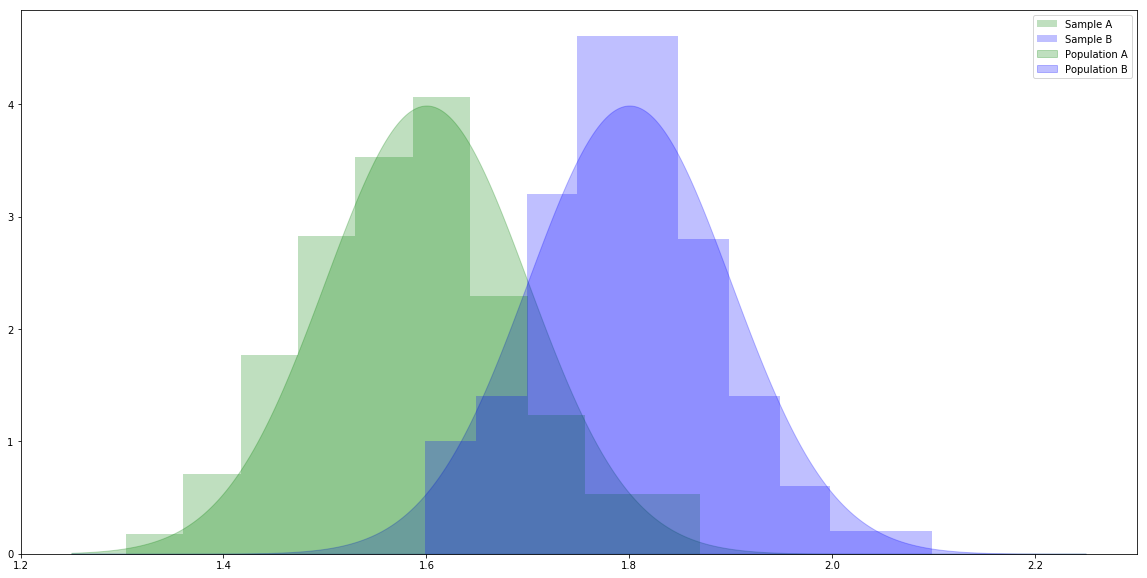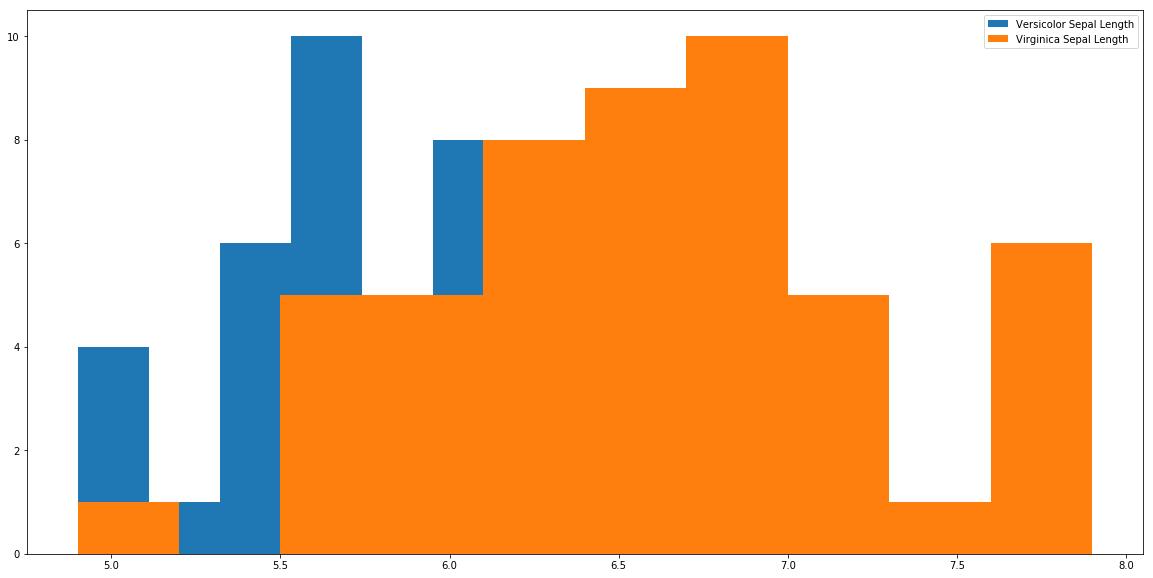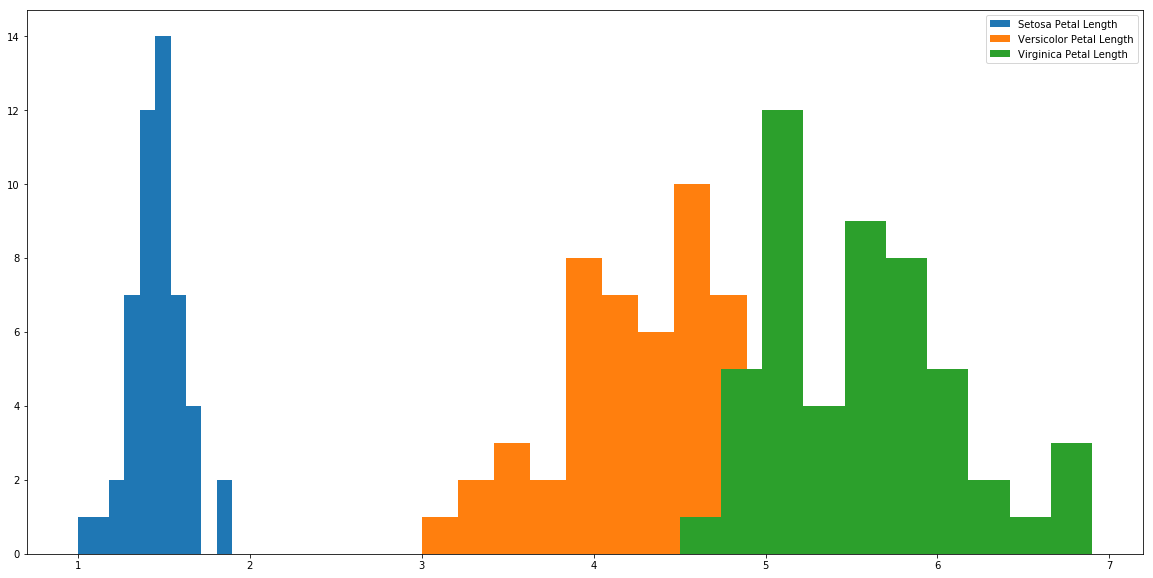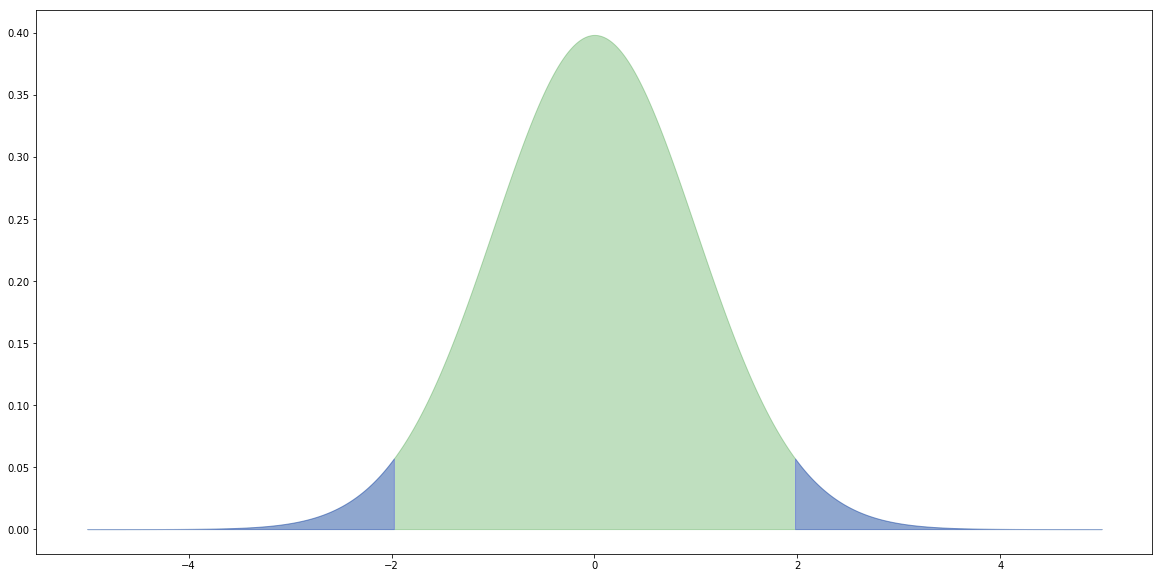# Analysis of variance (ANOVA)¶

In :
# For generating random variables.
import numpy as np
# For handling data.
import pandas as pd
# For plotting.
import matplotlib.pyplot as plt
# For t-tests and ANOVA.
import scipy.stats as stats

In :
# Make the plots bigger.
plt.rcParams['figure.figsize'] = (20.0, 10.0)

In :
# Set parameters for two populations.
popA = {'m': 1.6, 's': 0.1}
popB = {'m': 1.8, 's': 0.1}

# Create two samples, one from each population.
sampA = np.random.normal(popA['m'], popA['s'], 100)
sampB = np.random.normal(popB['m'], popB['s'], 100)

In :
# x values for plotting.
x = np.linspace(1.25, 2.25, 1000)

# The probability density functions (PDFs) for the two populations.
pdfA = stats.norm.pdf(x, popA['m'], popA['s'])
pdfB = stats.norm.pdf(x, popB['m'], popB['s'])

# Plot the population PDFs as shaded regions.
plt.fill_between(x, pdfA, color='g', alpha=0.25, label="Population A")
plt.fill_between(x, pdfB, color='b', alpha=0.25, label="Population B")

# Plot histograms of the two samples.
plt.hist(sampA, density=True, color='g', alpha=0.25, label="Sample A")
plt.hist(sampB, density=True, color='b', alpha=0.25, label="Sample B")

# Display a legend.
plt.legend()
plt.show()In :
# Calculate the independent samples t-statistic for the samples.
# We also get the probability of seeing samples at least as different as these given the population means are equal.
stats.ttest_ind(sampA, sampB)

Out:
Ttest_indResult(statistic=-15.515869408590305, pvalue=4.706971815270273e-36)

#### T-tests with Iris¶

In :
df = pd.read_csv('https://raw.githubusercontent.com/uiuc-cse/data-fa14/gh-pages/data/iris.csv')
df

Out:
sepal_length sepal_width petal_length petal_width species
0 5.1 3.5 1.4 0.2 setosa
1 4.9 3.0 1.4 0.2 setosa
2 4.7 3.2 1.3 0.2 setosa
3 4.6 3.1 1.5 0.2 setosa
4 5.0 3.6 1.4 0.2 setosa
5 5.4 3.9 1.7 0.4 setosa
6 4.6 3.4 1.4 0.3 setosa
7 5.0 3.4 1.5 0.2 setosa
8 4.4 2.9 1.4 0.2 setosa
9 4.9 3.1 1.5 0.1 setosa
10 5.4 3.7 1.5 0.2 setosa
11 4.8 3.4 1.6 0.2 setosa
12 4.8 3.0 1.4 0.1 setosa
13 4.3 3.0 1.1 0.1 setosa
14 5.8 4.0 1.2 0.2 setosa
15 5.7 4.4 1.5 0.4 setosa
16 5.4 3.9 1.3 0.4 setosa
17 5.1 3.5 1.4 0.3 setosa
18 5.7 3.8 1.7 0.3 setosa
19 5.1 3.8 1.5 0.3 setosa
20 5.4 3.4 1.7 0.2 setosa
21 5.1 3.7 1.5 0.4 setosa
22 4.6 3.6 1.0 0.2 setosa
23 5.1 3.3 1.7 0.5 setosa
24 4.8 3.4 1.9 0.2 setosa
25 5.0 3.0 1.6 0.2 setosa
26 5.0 3.4 1.6 0.4 setosa
27 5.2 3.5 1.5 0.2 setosa
28 5.2 3.4 1.4 0.2 setosa
29 4.7 3.2 1.6 0.2 setosa
... ... ... ... ... ...
120 6.9 3.2 5.7 2.3 virginica
121 5.6 2.8 4.9 2.0 virginica
122 7.7 2.8 6.7 2.0 virginica
123 6.3 2.7 4.9 1.8 virginica
124 6.7 3.3 5.7 2.1 virginica
125 7.2 3.2 6.0 1.8 virginica
126 6.2 2.8 4.8 1.8 virginica
127 6.1 3.0 4.9 1.8 virginica
128 6.4 2.8 5.6 2.1 virginica
129 7.2 3.0 5.8 1.6 virginica
130 7.4 2.8 6.1 1.9 virginica
131 7.9 3.8 6.4 2.0 virginica
132 6.4 2.8 5.6 2.2 virginica
133 6.3 2.8 5.1 1.5 virginica
134 6.1 2.6 5.6 1.4 virginica
135 7.7 3.0 6.1 2.3 virginica
136 6.3 3.4 5.6 2.4 virginica
137 6.4 3.1 5.5 1.8 virginica
138 6.0 3.0 4.8 1.8 virginica
139 6.9 3.1 5.4 2.1 virginica
140 6.7 3.1 5.6 2.4 virginica
141 6.9 3.1 5.1 2.3 virginica
142 5.8 2.7 5.1 1.9 virginica
143 6.8 3.2 5.9 2.3 virginica
144 6.7 3.3 5.7 2.5 virginica
145 6.7 3.0 5.2 2.3 virginica
146 6.3 2.5 5.0 1.9 virginica
147 6.5 3.0 5.2 2.0 virginica
148 6.2 3.4 5.4 2.3 virginica
149 5.9 3.0 5.1 1.8 virginica

150 rows × 5 columns

In :
s = df[df['species'] == 'setosa']
r = df[df['species'] == 'versicolor']
a = df[df['species'] == 'virginica']

In :
print(stats.ttest_ind(s['petal_length'], r['petal_length']))
print(stats.ttest_ind(s['petal_length'], a['petal_length']))
print(stats.ttest_ind(r['petal_length'], a['petal_length']))

print(stats.ttest_ind(s['petal_width'], r['petal_width']))
print(stats.ttest_ind(s['petal_width'], a['petal_width']))
print(stats.ttest_ind(r['petal_width'], a['petal_width']))

print(stats.ttest_ind(s['sepal_length'], r['sepal_length']))
print(stats.ttest_ind(s['sepal_length'], a['sepal_length']))
print(stats.ttest_ind(r['sepal_length'], a['sepal_length']))

print(stats.ttest_ind(s['sepal_width'], r['sepal_width']))
print(stats.ttest_ind(s['sepal_width'], a['sepal_width']))
print(stats.ttest_ind(r['sepal_width'], a['sepal_width']))

Ttest_indResult(statistic=-39.46866259397272, pvalue=5.717463758170621e-62)
Ttest_indResult(statistic=-49.965703359355636, pvalue=1.5641224158883576e-71)
Ttest_indResult(statistic=-12.603779441384985, pvalue=3.1788195478061495e-22)
Ttest_indResult(statistic=-34.01237858829048, pvalue=4.589080615710866e-56)
Ttest_indResult(statistic=-42.738229672411165, pvalue=3.582719502316063e-65)
Ttest_indResult(statistic=-14.625367047410148, pvalue=2.2304090710248333e-26)
Ttest_indResult(statistic=-10.52098626754911, pvalue=8.985235037487077e-18)
Ttest_indResult(statistic=-15.386195820079404, pvalue=6.892546060674059e-28)
Ttest_indResult(statistic=-5.629165259719801, pvalue=1.7248563024547942e-07)
Ttest_indResult(statistic=9.282772555558111, pvalue=4.362239016010214e-15)
Ttest_indResult(statistic=6.289384996672061, pvalue=8.916634067006443e-09)
Ttest_indResult(statistic=-3.2057607502218186, pvalue=0.0018191004238894803)


#### Problems with t-tests¶

Website: Multiple t tests and Type I error

http://grants.hhp.coe.uh.edu/doconnor/PEP6305/Multiple%20t%20tests.htm

Webpage about multiple t tests and Type I errors.

Wikipedia: Multiple Comparisons Problem

https://en.wikipedia.org/wiki/Multiple_comparisons_problem

In :
plt.hist(r['sepal_length'], label='Versicolor Sepal Length')
plt.hist(a['sepal_length'], label='Virginica Sepal Length')

plt.legend()

plt.show()In :
1- ((0.95)**12)

Out:
0.45963991233736334
In :
stats.f_oneway(s['petal_length'], r['petal_length'], a['petal_length'])

Out:
F_onewayResult(statistic=1179.0343277002194, pvalue=3.0519758018278374e-91)
In :
plt.hist(s['petal_length'], label='Setosa Petal Length')
plt.hist(r['petal_length'], label='Versicolor Petal Length')
plt.hist(a['petal_length'], label='Virginica Petal Length')

plt.legend()

plt.show()When performing an independent sample t-test we assume that there is a given difference between the means of two populations, usually a difference of zero.

We then look at the samples to investigate how different they are, calculating their t-statistic.

We then ask, given the hypothesised difference (usually zero) what was the probability of seeing a t-statistic at least this extreme.

If it's too extreme (say, less that 5% chance of seeing it) then we say our hypothesis about the difference must be wrong.

### Errors¶

Of course, we might, by random chance, see a t-statistic that extreme.

We might reject the hypothesis incorrectly - the populations might have the hypothesised difference and the samples just randomly happened to be as different as they are.

We call that a Type I error.

We also might not reject the hypothesis when it's not true - that's a Type II error.### Calculating the t-statistic¶

Note that we are using the calculations for two samples, with equal variances, and possibly different sample sizes.

$$t={\frac {{\bar {X}}_{1}-{\bar {X}}_{2}}{s_{p}\cdot {\sqrt {{\frac {1}{n_{1}}}+{\frac {1}{n_{2}}}}}}}}$$$$s_{p}={\sqrt {\frac {\left(n_{1}-1\right)s_{X_{1}}^{2}+\left(n_{2}-1\right)s_{X_{2}}^{2}}{n_{1}+n_{2}-2}}}}$$$$s^{2}={\frac {1}{n-1}}\sum _{i=1}^{n}\left(Y_{i}-{\overline {Y}}\right)^{2}}$$
In :
# Count the samples.
nA = float(len(sampA))
nB = float(len(sampB))

# Calculate the means.
mA = sampA.sum() / nA
mB = sampB.sum() / nB

# Sample variances.
varA = ((sampA - mA)**2).sum() / (nA - 1.0)
varB = ((sampB - mB)**2).sum() / (nB - 1.0)

# Pooled standard deviation.
sp = np.sqrt(((nA - 1.0) * varA + (nB - 1.0) * varB) / (nA + nB - 2.0))

# t-statistic
t = (mA - mB) / (sp * np.sqrt((1.0 / nA) + (1.0 / nB)))

print(f"Mean of sample A:       {mA:8.4f}")
print(f"Mean of sample B:       {mB:8.4f}")
print(f"Size of sample A:       {nA:8.4f}")
print(f"Size of sample B:       {nB:8.4f}")
print(f"Variance of sample A:   {varA:8.4f}")
print(f"Variance of sample B:   {varB:8.4f}")
print(f"Pooled std dev:         {sp:8.4f}")
print(f"t-statistic:            {t:8.4f}")

Mean of sample A:         1.5857
Mean of sample B:         1.8002
Size of sample A:       100.0000
Size of sample B:       100.0000
Variance of sample A:     0.0111
Variance of sample B:     0.0080
Pooled std dev:           0.0978
t-statistic:            -15.5159


#### Critical values¶

For a two-tail test (e.g. $H_0$: the means are equal) we reject the null hypothesis $H_0$ if the value of the t-statistic from the samples is further away from zero than the t-statistic at the ($0.5 / 2.0 =$) $0.025$ level.

In :
# x values for plotting.
x = np.linspace(-5.0, 5.0, 1000)

# The probability density functions (PDFs) for the t distribution.
# The number of degrees of freedom is (nA + nB - 2).
pdf = stats.t.pdf(x, (nA + nB - 2.0))

# Create a dataframe from x and pdf.
df = pd.DataFrame({'x': x, 'y': pdf})

# Plot the overall distribution.
plt.fill_between(df['x'], df['y'], color='g', alpha=0.25)

# Plot the values more extreme than our |t|.
crit = np.abs(stats.t.ppf(0.975, nA + nB - 2.0))
tail1 = df[df['x'] >=  crit]
tail2 = df[df['x'] <= -crit]
plt.fill_between(tail1['x'], tail1['y'], color='b', alpha=0.25)
plt.fill_between(tail2['x'], tail2['y'], color='b', alpha=0.25)

print(crit)

plt.show()

1.9720174778338955At a level of 0.05, there's a one in twenty chance that we incorrectly reject the null hypotheses.

In :
m = 10.0
s = 1.0
t = 1000
a = 0.05

sum([1 if stats.ttest_ind(np.random.normal(m, s, 100), np.random.normal(m, s, 100)) <= a else 0 for i in range(t)])

Out:
33